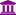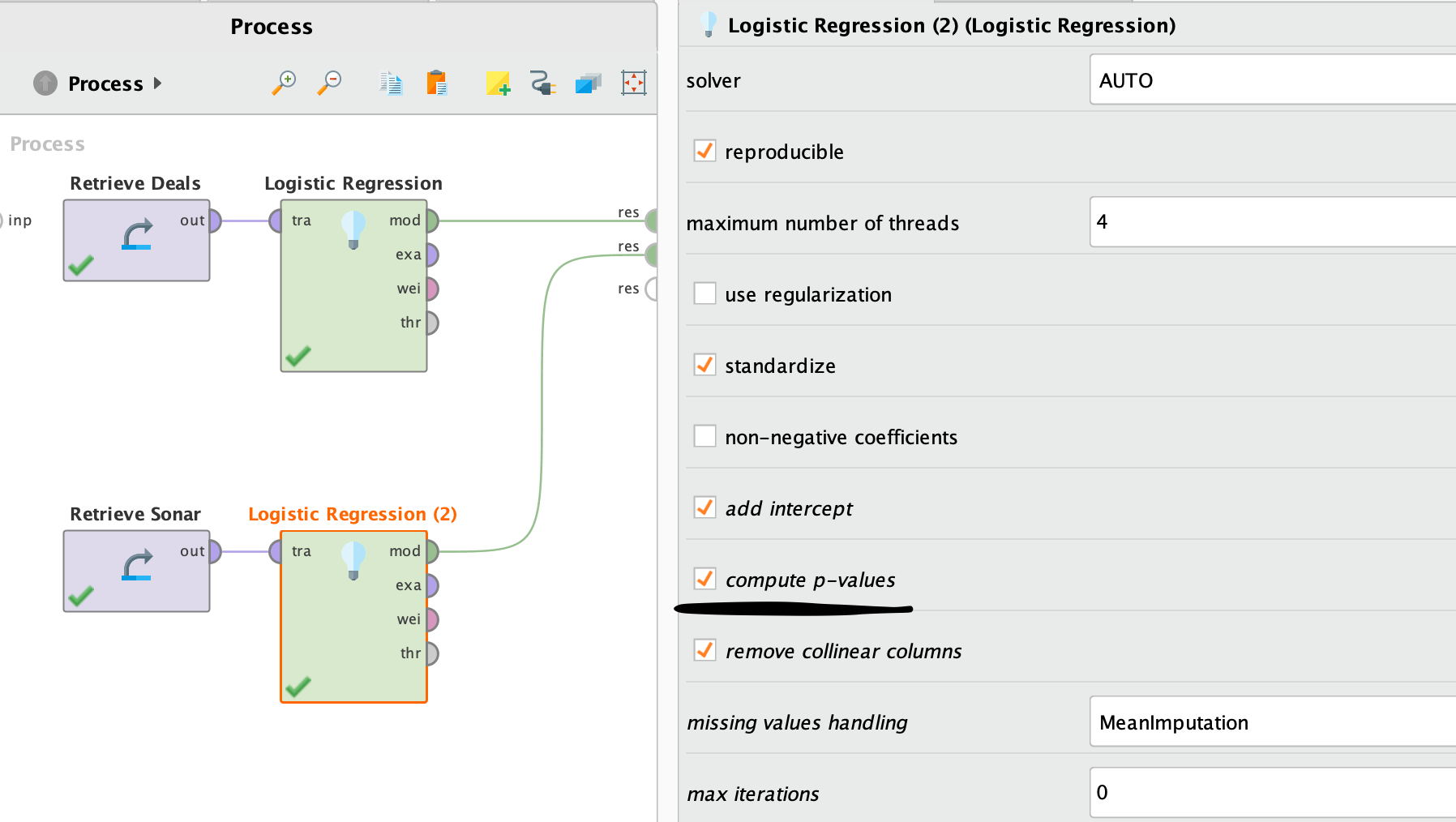# Logistic Regression Wald test

Member, University Professor Posts: 5University Professor
edited March 1 in Help
I cannot find which performance operator gives me the wald test for logistic regression
Tagged:

• Administrator, Employee, RapidMiner Certified Analyst, RapidMiner Certified Expert, Member Posts: 273RM Data Scientist
Hi @Mori111,

Do you want to compute p-value for the significance of the factors in Logistic Regression? Just check the box and enable it in GLM or Log Regression operator.```<?xml version="1.0" encoding="UTF-8"?><process version="9.6.000">
<context>
<input/>
<output/>
<macros/>
</context>
<operator activated="true" class="process" compatibility="9.4.000" expanded="true" name="Process" origin="GENERATED_TUTORIAL">
<parameter key="logverbosity" value="init"/>
<parameter key="random_seed" value="2001"/>
<parameter key="send_mail" value="never"/>
<parameter key="process_duration_for_mail" value="30"/>
<parameter key="encoding" value="SYSTEM"/>
<process expanded="true">
<operator activated="true" class="retrieve" compatibility="9.6.000" expanded="true" height="68" name="Retrieve Deals" origin="GENERATED_TUTORIAL" width="90" x="45" y="34">
<parameter key="repository_entry" value="//Samples/data/Deals"/>
</operator>
<operator activated="true" class="h2o:logistic_regression" compatibility="9.3.001" expanded="true" height="124" name="Logistic Regression" origin="GENERATED_TUTORIAL" width="90" x="179" y="34">
<parameter key="solver" value="AUTO"/>
<parameter key="reproducible" value="true"/>
<parameter key="use_regularization" value="false"/>
<parameter key="lambda_search" value="false"/>
<parameter key="number_of_lambdas" value="0"/>
<parameter key="lambda_min_ratio" value="0.0"/>
<parameter key="early_stopping" value="true"/>
<parameter key="stopping_rounds" value="3"/>
<parameter key="stopping_tolerance" value="0.001"/>
<parameter key="standardize" value="true"/>
<parameter key="non-negative_coefficients" value="false"/>
<parameter key="compute_p-values" value="true"/>
<parameter key="remove_collinear_columns" value="true"/>
<parameter key="missing_values_handling" value="MeanImputation"/>
<parameter key="max_iterations" value="0"/>
<parameter key="max_runtime_seconds" value="0"/>
</operator>
<operator activated="true" class="retrieve" compatibility="9.6.000" expanded="true" height="68" name="Retrieve Sonar" width="90" x="45" y="238">
<parameter key="repository_entry" value="//Samples/data/Sonar"/>
</operator>
<operator activated="true" class="h2o:logistic_regression" compatibility="9.3.001" expanded="true" height="124" name="Logistic Regression (2)" origin="GENERATED_TUTORIAL" width="90" x="179" y="238">
<parameter key="solver" value="AUTO"/>
<parameter key="reproducible" value="true"/>
<parameter key="use_regularization" value="false"/>
<parameter key="lambda_search" value="false"/>
<parameter key="number_of_lambdas" value="0"/>
<parameter key="lambda_min_ratio" value="0.0"/>
<parameter key="early_stopping" value="true"/>
<parameter key="stopping_rounds" value="3"/>
<parameter key="stopping_tolerance" value="0.001"/>
<parameter key="standardize" value="true"/>
<parameter key="non-negative_coefficients" value="false"/>
<parameter key="compute_p-values" value="true"/>
<parameter key="remove_collinear_columns" value="true"/>
<parameter key="missing_values_handling" value="MeanImputation"/>
<parameter key="max_iterations" value="0"/>
<parameter key="max_runtime_seconds" value="0"/>
</operator>
<connect from_op="Retrieve Deals" from_port="output" to_op="Logistic Regression" to_port="training set"/>
<connect from_op="Logistic Regression" from_port="model" to_port="result 1"/>
<connect from_op="Retrieve Sonar" from_port="output" to_op="Logistic Regression (2)" to_port="training set"/>
<connect from_op="Logistic Regression (2)" from_port="model" to_port="result 2"/>
<portSpacing port="source_input 1" spacing="0"/>
<portSpacing port="sink_result 1" spacing="0"/>
<portSpacing port="sink_result 2" spacing="0"/>
<portSpacing port="sink_result 3" spacing="0"/>
</process>
</operator>
</process>
```

Cheers,
YY
• Member, University Professor Posts: 5University Professor